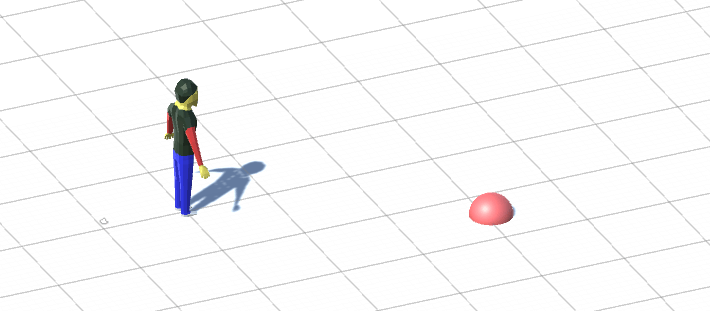# Use math to solve problems in Unity with C#

## 3. Turn left or right to reach a waypoint?

If you have a character following a series of waypoints, how can you tell if the character should turn left or right to reach the waypoint? We can of course always turn left, but we want to turn the smallest possible distance. This is the basic scene:To solve this problem we are going to use the Cross Product. The basic idea is that we have two vectors: one going from you to the waypoint and we also know in which direction you are facing. Now we can use the fact that the direction of the cross product is determined by whether the first vector is to the left or to the right of the second vector. Then we can use the dot product to determine the direction of this cross product, in a similar way as in the first part of this tutorial series. This is how the code looks like:

```using UnityEngine;
using System.Collections;

namespace LinearAlgebra
{
//Figure out if you should turn left or right to reach a waypoint
public class LeftOrRight : MonoBehaviour
{
public Transform youTrans;
public Transform wayPointTrans;

void Update()
{
//The direction you are facing
Vector3 youDir = youTrans.forward;

//The direction from you to the waypoint
Vector3 waypointDir = wayPointTrans.position - youTrans.position;

//The cross product between these vectors
Vector3 crossProduct = Vector3.Cross(youDir, waypointDir);

//The dot product between the your up vector and the cross product
//This can be said to be a volume that can be negative
float dotProduct = Vector3.Dot(crossProduct, youTrans.up);

//Now we can decide if we should turn left or right
if (dotProduct > 0f)
{
Debug.Log("Turn right");
}
else
{
Debug.Log("Turn left");
}
}
}
}
```

After finishing this section of the tutorial, someone said that there's a more efficient way to determine if we should turn left or right. You can actually solve this problem by using just the Dot Product. So this tutorial will also show that there may be a more efficient way to solve your problems. This is the code for the more efficient version:

```//The right direction of the direction you are facing
Vector3 youDir = youTrans.right;

//The direction from you to the waypoint
Vector3 waypointDir = wayPointTrans.position - youTrans.position;

//The dot product between the vectors
float dotProduct = Vector3.Dot(youDir, waypointDir);

//Now we can decide if we should turn left or right
if (dotProduct > 0f)
{
Debug.Log("Turn right");
}
else
{
Debug.Log("Turn left");
}
```

### Turn left or right to reach a direction?

In the above examples we wanted to reach a position. But what if we want to know if we should turn left or right to reach a direction? That's actually even simpler!

```//The right direction of the direction you are facing
Vector3 youDir = youTrans.right;

//The direction you want to reach by turning left or right
//Here we want to reach the forward direction of the waypoint
Vector3 waypointDir = wayPointTrans.forward;

//The dot product between the vectors
float dotProduct = Vector3.Dot(youDir, waypointDir);

//Now we can decide if we should turn left or right
if (dotProduct > 0f)
{
Debug.Log("Turn right");
}
else
{
Debug.Log("Turn left");
}
```